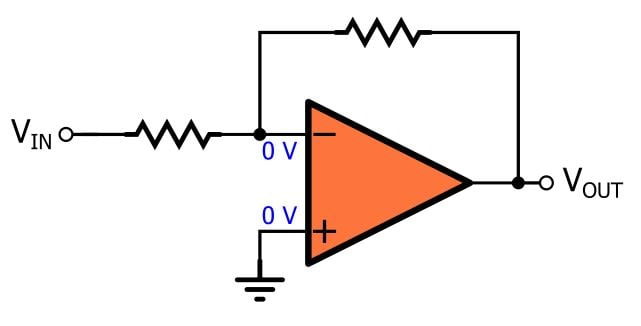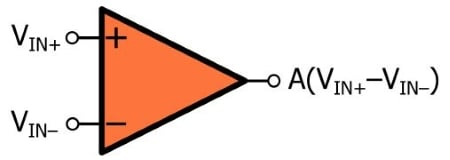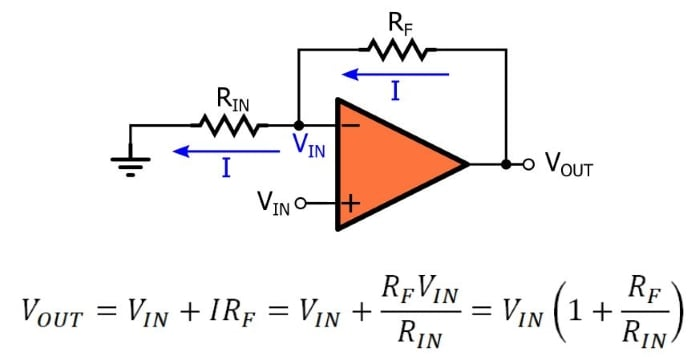Technical Article

# Understanding the Virtual Short in Op Amp Circuits

November 05, 2018 by Robert Keim

## This article explains and discusses an important simplification technique used in op amp analysis.

This article explains and discusses an important simplification technique used in op amp analysis.

### Related Information

For those who are seeking genuine expertise in real-life circuit design, gaining a more thorough understanding of op amp functionality and implementation is, in my opinion, time well spent. These components are extremely common, and rightly so—they are straightforward, versatile devices that provide the required functionality in a seemingly endless list of applications.

The analysis and design of op amp circuits is an interesting thing, for the following reason: we analyze op amps with the help of simplifying assumptions that are clearly false, yet we rarely experience serious problems caused by the differences between the real device and the idealized device on which the assumptions are based.

### The Virtual Short

One of these assumptions is called the virtual short circuit, or just virtual short. Actually, this is not one of the fundamental characteristics of an ideal op amp. Rather, the virtual short is a theoretical situation that arises from one of the fundamental characteristics of an ideal op amp, namely, infinite open-loop gain.

Let’s imagine that we have an op amp connected as an inverting amplifier. As is almost always the case with op amp circuits, the functionality is based on the use of negative feedback.The standard method of obtaining an expression for the gain of this circuit is to assume that the voltage at the noninverting input terminal (VIN+) is equal to the voltage at the inverting terminal (VIN–). Since the noninverting input terminal is grounded, VIN+ = 0 V and thus VIN– = 0 V. But why? Why can we assume that two different voltages are the same?

#### Infinite Gain

We can assume that two different voltages are the same because in reality the difference between these voltages is very small, and the difference between the voltages is very small because the gain is very large. Consider the following diagram and equation:$$V_{OUT}=A\times(V_{IN+}-V_{IN-})$$

An op amp is a differential amplifier. It produces an output voltage by applying an open-loop gain (denoted by A) to the difference between the voltage at the noninverting input terminal and the voltage at the inverting input terminal. If we rearrange this equation so that the difference voltage is separated from the gain, we have the following:

$$\frac{V_{OUT}}{A}=V_{IN+}-V_{IN-}$$

Notice what happens as the open-loop gain increases (for a given VOUT): the difference voltage decreases. As gain approaches infinity, the difference voltage approaches zero. In other words, if the gain is infinite, VIN+ must be equal to VIN–, and this is the virtual short.

#### Finite Gain

The impossibility of building an infinite-gain amplifier doesn’t nullify the practical value of the virtual short assumption. Why? Because “virtual short” is simply another way of saying that there is zero difference between the op amp’s two input voltages, and in real circuits the difference is “close enough” to zero. The open-loop gain of real op amps can be greater than 100 dB. That’s an output-to-input ratio of at least 100,000. Let’s say we have an op amp with A = 100 dB, and it’s producing an output voltage of 2.5 V.

$$V_{IN+}-V_{IN-}=\frac{2.5\ V}{100,000}=0.000025\ V$$

That’s 25 µV. When the open-loop gain is high enough to produce a (very) small difference voltage, the virtual short circuit is reliable as a practical design tool, despite the fact that it is unrealistic. And actually, it’s not only unrealistic. It’s downright paradoxical.

### Pondering the Details

I don’t like it when someone tells me that applying a gain to a 0 V signal produces a 2.5 V output. I learned a long time ago that zero times something equals zero. The ideal op amp is a differential amplifier, and its input signal (i.e., VIN+–VIN–) is zero. Thus, the ideal op amp produces an output voltage of zero under all conditions ... that doesn’t seem like a very useful device.

Of course, this is where the assumption breaks down. You can’t use the virtual short to determine VOUT based on the normal open-loop functionality of the op amp. Rather, the virtual short is a tool that we use when the op amp is implemented within the context of negative feedback. For example, when we’re analyzing a standard noninverting amplifier, the virtual short assumption (in conjunction with the zero-input-current assumption) allows us to derive an expression for the closed-loop gain as if the op amp is not even there.When I look at this circuit, my mind naturally ignores the triangle. The gain of the op amp is wrapped up in the virtual short assumption, and beyond that, the triangle is like a stone dropped into a stream. The current just flows around it.

#### Is the Input an Input or an Output?

If you ground an op amp’s inverting input terminal and apply a 1 V signal to the noninverting input terminal, the output will saturate at (or near) the positive rail and the difference between VIN+ and VIN– will be 1 V. There is nothing surprising here; the device’s high open-loop gain drives the output to the maximum output voltage, and the input voltage stays at 1 V.

When we start to talk about the virtual short, though, the situation is less straightforward. It almost seems as though the op amp is using its high gain to force the input voltages into compliance. In the inverting configuration, for example, one input is grounded and the other receives the input signal, but then the op amp decides that those two voltages should be the same. What’s really going on here?

Again, the virtual short assumption is something that exists within the context of a negative-feedback amplifier. In the inverting configuration, the inverting input terminal is connected not only to the input signal (through a resistor) but also to the output terminal (through a resistor). The output voltage affects the voltage at the inverting input terminal, and the gain of the op amp affects the output voltage, and this leads to my final statements regarding what the virtual short really is:

• The presence of a negative feedback network establishes an input-to-output/output-to-input relationship;
• the difference between VIN+ and VIN– must be consistent with the closed-loop gain imposed by the feedback network;
• thus, the circuit naturally creates a situation in which the difference between the inverting and noninverting input voltages is very small, because the only way to produce a typical output voltage from an extremely-high-gain difference amplifier is to have a very small difference voltage.

### Conclusion

I hope that this discussion has helped you to more thoroughly understand the virtual short assumption, which was on my mind recently when I wrote an article on charge amplifiers for piezoelectric sensors—the virtual short (in this case a virtual ground) allows the output signal to be unaffected by changes in cable capacitance. If you have any additional insights on this topic, feel free to share your thoughts in the comments section below.•brianrho November 10, 2018

Neat article! Though this line: “I learned a long time ago that zero times something equals zero” is off, I think. When said ‘something’ is infinity, the result is “undefined”, could very well be 2.5 V 😉

Like.
• B
btremaine November 11, 2018
More precisely use l'hospital's rule ;) the derivative of the numerator evaluated at 0 divided by the derivative of the denominator evaluated at 0.
Like.
•brianrho November 10, 2018

Neat article! Though this line: “I learned a long time ago that zero times something equals zero” is off, I think. When said ‘something’ is infinity, the result is “undefined”, could very well be 2.5 V 😉

Like.
•RK37 January 30, 2019
Infinity is not really a number. It's a concept. Infinity can be incorporated into mathematical study, but when we're dealing with basic calculations, any quantity multiplied by zero is zero.
Like.
• L
LambertJ January 25, 2019

Good article about an elusive subject. Our op amp course is taught in conjunction with a course on continuous control (P, PI and PID) concepts. The two concepts, op amps and proportional control, are very similar and mutually suppotive if one assumes the non- inverting input is the set point and the feedback voltage to the inverting input is the process variable. I’ve found that using realistic open-loop voltage gains of approx 200k and determining the “droop” or residual error byproduct of proportional control will yield a difference between inputs, Vid = Vnon - Vinv, of microvolts which is essentially zero. Even better, build a simple negative feedback amplifier and measure the difference between op amp inputs. As long as Vout is not equal to either rail the input difference will be within a few millivolts of zero. Thanks - JL.

Like.
•RK37 January 30, 2019
Interesting comment. Thanks for contributing to the discussion.
Like.